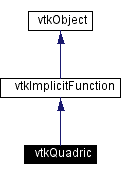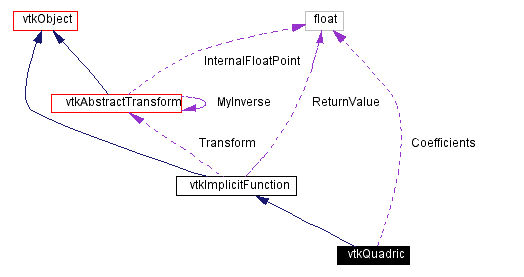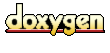Main Page   Class Hierarchy   Alphabetical List   Compound List   File List   Compound Members   File Members   Related Pages

`#include <vtkQuadric.h>`[legend][legend]
List of all members.

## Public Methods

virtual const char * GetClassName ()
virtual int IsA (const char *type)
void PrintSelf (ostream &os, vtkIndent indent)
float EvaluateFunction (float x)
float EvaluateFunction (float x, float y, float z)
void EvaluateGradient (float x, float g)
void SetCoefficients (float a)
void SetCoefficients (float a0, float a1, float a2, float a3, float a4, float a5, float a6, float a7, float a8, float a9)
virtual float * GetCoefficients ()
virtual void GetCoefficients (float data)

## Static Public Methods

int IsTypeOf (const char *type)

## Protected Attributes

float Coefficients 

## Detailed Description

Date:
2000/12/10 20:08:17
Revision:
1.2

vtkQuadric evaluates the quadric function F(x,y,z) = a0*x^2 + a1*y^2 + a2*z^2 + a3*x*y + a4*y*z + a5*x*z + a6*x + a7*y + a8*z + a9. vtkQuadric is a concrete implementation of vtkImplicitFunction.

Examples:

Definition at line 59 of file vtkQuadric.h.

## Constructor & Destructor Documentation

 vtkQuadric::vtkQuadric ( ) ` [protected]`

 vtkQuadric::~vtkQuadric ( ) ` [inline, protected]`
 Definition at line 84 of file vtkQuadric.h.

 vtkQuadric::vtkQuadric ( const vtkQuadric & ) ` [inline, protected]`
 Definition at line 85 of file vtkQuadric.h.

## Member Function Documentation

 virtual const char* vtkQuadric::GetClassName ( ) ` [virtual]`
 Return the class name as a string. This method is defined in all subclasses of vtkObject with the vtkTypeMacro found in vtkSetGet.h. Reimplemented from vtkImplicitFunction.

 int vtkQuadric::IsTypeOf ( const char * type ) ` [static]`
 Return 1 if this class type is the same type of (or a subclass of) the named class. Returns 0 otherwise. This method works in combination with vtkTypeMacro found in vtkSetGet.h. Reimplemented from vtkImplicitFunction.

 virtual int vtkQuadric::IsA ( const char * type ) ` [virtual]`
 Return 1 if this class is the same type of (or a subclass of) the named class. Returns 0 otherwise. This method works in combination with vtkTypeMacro found in vtkSetGet.h. Reimplemented from vtkImplicitFunction.

 vtkQuadric* vtkQuadric::SafeDownCast ( vtkObject * o ) ` [static]`
 Will cast the supplied object to vtkObject* is this is a safe operation (i.e., a safe downcast); otherwise NULL is returned. This method is defined in all subclasses of vtkObject with the vtkTypeMacro found in vtkSetGet.h. Reimplemented from vtkImplicitFunction.

 void vtkQuadric::PrintSelf ( ostream & os, vtkIndent indent ) ` [virtual]`
 Methods invoked by print to print information about the object including superclasses. Typically not called by the user (use Print() instead) but used in the hierarchical print process to combine the output of several classes. Reimplemented from vtkImplicitFunction.

 vtkQuadric* vtkQuadric::New ( ) ` [static]`
 Construct quadric with all coefficients = 1. Reimplemented from vtkObject.

 float vtkQuadric::EvaluateFunction ( float x ) ` [virtual]`
 Evaluate quadric equation. Reimplemented from vtkImplicitFunction.

 float vtkQuadric::EvaluateFunction ( float x, float y, float z ) ` [inline]`
 Reimplemented from vtkImplicitFunction. Definition at line 70 of file vtkQuadric.h.

 void vtkQuadric::EvaluateGradient ( float x, float g ) ` [virtual]`

 void vtkQuadric::SetCoefficients ( float a )
 Set / get the 10 coefficients of the quadric equation.

 void vtkQuadric::SetCoefficients ( float a0, float a1, float a2, float a3, float a4, float a5, float a6, float a7, float a8, float a9 )

 virtual float* vtkQuadric::GetCoefficients ( ) ` [virtual]`

 virtual void vtkQuadric::GetCoefficients ( float data ) ` [virtual]`

 void vtkQuadric::operator= ( const vtkQuadric & ) ` [inline, protected]`
 Definition at line 86 of file vtkQuadric.h.

## Member Data Documentation

 float vtkQuadric::Coefficients` [protected]`
 Definition at line 88 of file vtkQuadric.h.

The documentation for this class was generated from the following file:

Generated on Wed Nov 21 12:58:15 2001 for VTK by1.2.11.1 written by Dimitri van Heesch, © 1997-2001